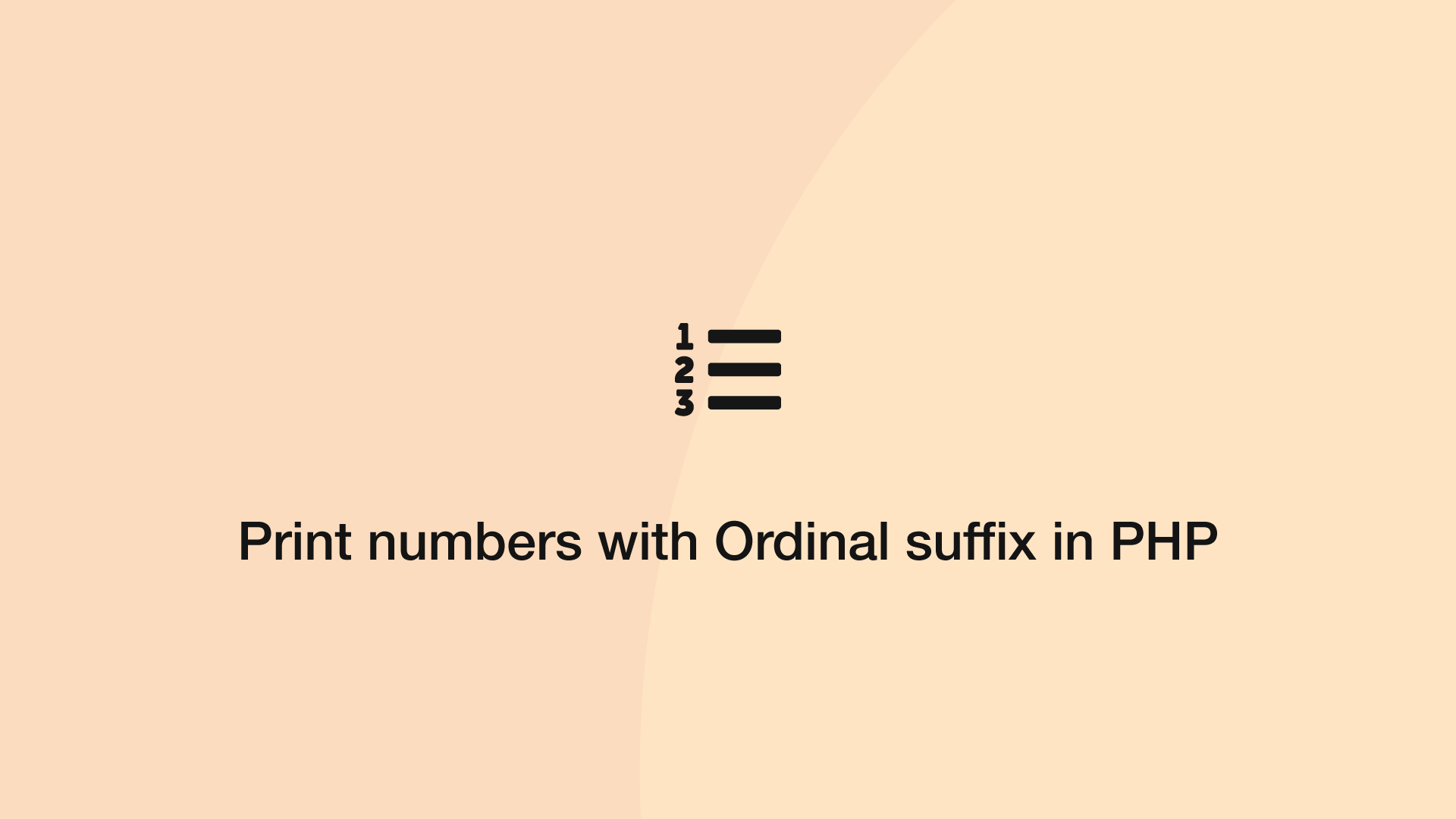# How to Print Numbers with Ordinal Suffix in PHPOrdinal suffixes are words that represent the rank of a number. In this tutorial, we will learn how to add ordinal numerals to the end of each number dynamically using PHP.

The first step is to create an array of ordinal suffixes and store them in a variable.

``````\$ordinals = ['th','st','nd','rd','th','th','th','th','th','th'];
``````

Now we can assign an appropriate number to a numeral.

``````for (\$i=1; \$i < 22; \$i++) {
print_r(\$i . ((\$i%100) >= 11 && (\$i%100) <= 13 ? 'th' : \$ordinals[\$i % 10]) . "<br>");
}
``````
``````1st
2nd
3rd
4th
5th
6th
7th
8th
9th
10th
11th
12th
13th
14th
15th
16th
17th
18th
19th
20th
21st
``````

The only numbers that disrupt the ordinal suffix pattern are 11,12 and 13 which end in "th". The above example code looks for the 11th, 12th and 13th number in every 100 and uses a "th" suffix, otherwise, the appropriate ordinal is picked from the array.

#### Related Tutorials### How to Encode, Decode and Use JSON Data in PHP

September 06, 2020### How to Create and Use Lists (Arrays) in Python

September 01, 2020### How to Echo or Print an Array in PHP

June 04, 2021### PHP DateTime - Create, Format and Compare Dates with PHP

September 04, 2020### How to Parse JSON Data in Python (Read and Write)

September 18, 2020June 29, 2020